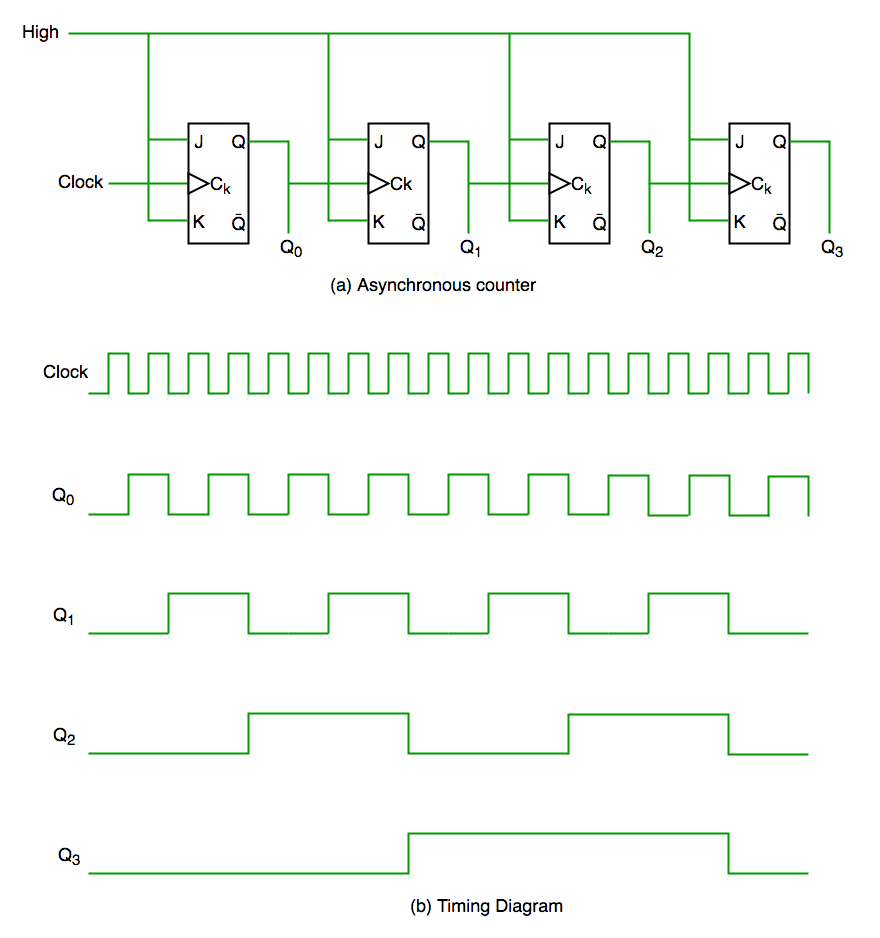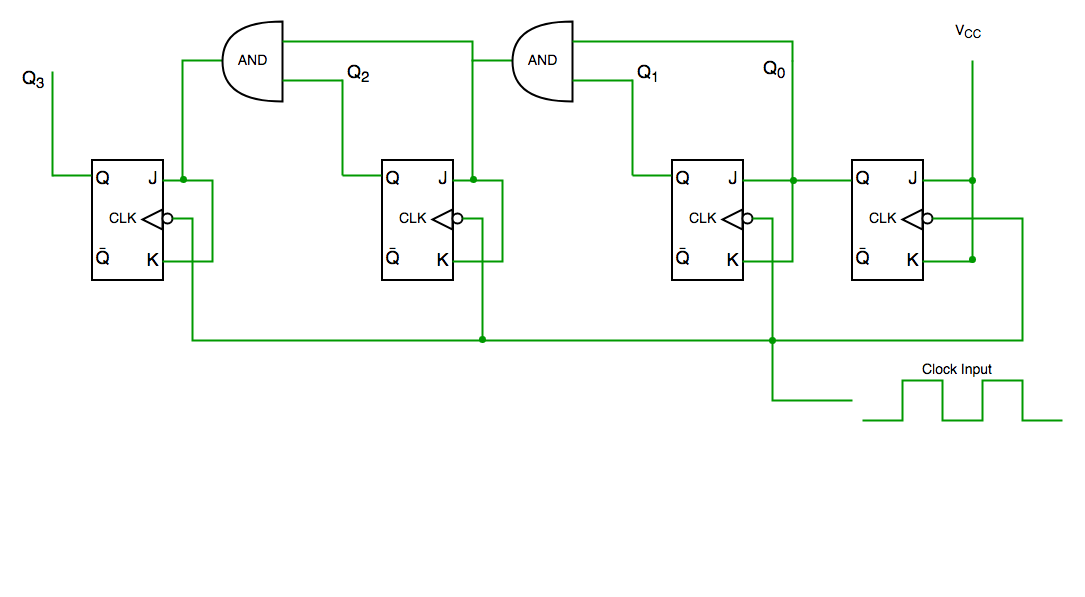Open In App

# Differences between Synchronous and Asynchronous Counter

There are two types of counters in digital logic circuit that are used to count the numbers of bits and these types depends upon the clock pulse applied to the flip flops.

1. Asynchronous Counter:

These are the counters in which we do not use universal clock, main clock is only applied to the first flip flop and then for rest of flip flops the output of previous flip flop is taken as a clock.ASYNCHRONOUS COUNTER

2. Synchronous Counter:

These are the counters in which we use a universal clock that is common to all flip flops. The Circuit diagram of Synchronous Counter is given Below:SYNCHRONOUS COUNTER

Difference Table:

Let’s see the difference between these two counters: